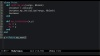Module 1, Topic 7
In Progress

# Constructors

Module Progress
0% CompleteIf you came to Ruby from a language like Java, JavaScript, or C#, one of the differences you probably noticed early on was how Ruby handles creating objects. In most modern OO languages, creating an instance looks something like this, with the keyword `new` followed by the name of the class:

``````new Point(3,5);
``````

Whereas in Ruby, the `new` is a method called on the class:

``````Point.new(3,5)
``````

And then things get stranger still: whereas in most other languages, a constructor is a method with the same name as the class, in Ruby we define our constructor by writing a method called "initialize".

``````class Point
def initialize(x,y)
@x = x
@y = y
end
end
``````

Today, let's explore how Ruby constructs new objects, and how we can customize the process to our own ends.

The easiest way to understand object construction in Ruby is to rewrite it ourselves.

We'll start with a class-level method called `.my_new`.

``````class Point
def self.my_new(x,y)
end
end
``````

To construct a new instance, Ruby needs to do three things:

1. Allocate a new, empty object of the given class.
2. Run any specialized initialization code defined for the class; and
3. Return the initialized instance.

For step one, allocating a new, empty object, we use a built-in method called, appropriately enough, `allocate`.

``````class Point
def self.my_new(x,y)
instance = allocate
end
end
``````

Remember that we are defining a class method here, so the object we are sending `#allocate` to is the `Point` class itself.

Step two is running specialized initialization for the object. In this case, we want to set two instance variables, `@x` and `@y`. But setting instance variables from outside the instance is pretty painful:

``````class Point
def self.my_new(x,y)
instance = allocate
instance.instance_variable_set(:@x, x)
instance.instance_variable_set(:@x, y)
end
end
``````

It would be easier if we could delegate that task to an instance method on the new object. We'll call the instance method `#my_initialize`.

``````class Point
def self.my_new(x,y)
instance = allocate
instance.my_initialize(x,y)
end

def my_initialize(x,y)
@x = x
@y = y
end
end
``````

It occurs to us that the Point initializer might not always take just two arguments. What if we added names for the points? Or a third dimension? We don't want to have to change the list of parameters in three different places. So we generalize the constructor method to take any arguments, and simply pass them along to the initializer instance method as-is using the splat operator.

While we're at it, we also arrange for any block provided to the constructor to be passed along as well, using an & parameter.

``````class Point
def self.my_new(*args, &block)
instance = allocate
instance.my_initialize(*args, &block)
end

def my_initialize(x,y)
@x = x
@y = y
end
end
``````

The third step in object construction is to return the initialized object. We update our constructor method to return the new instance.

``````class Point
def self.my_new(*args, &block)
instance = allocate
instance.my_initialize(*args, &block)
instance
end
# ...
end
``````

And there we have it: a complete reimplementation of how Ruby's own `.new` method works. We can try it out and see that it works:

``````p = Point.my_new(3,5)
``````

When we look at our implementation of `.my_new`, we can see that the call to `#my_initialize` functions as a kind of callback: it handles class-specific instance initialization only, while the class-level `.my_new` method takes care of allocating an object and making sure that the new object is returned after initialization.

The code we've just written is exactly how Ruby's own `.new` and `#initialize` work. And when we realize this, we realize we can make our own constructors to serve different purposes. For instance, consider a `Point` class which may be instantiated with either polar or Cartesian coordinates. Both coordinate systems use two numbers to locate a point. How can the initializer figure out which coordinate system its arguments belong to?

We can make the coordinate system explicit by defining specialized constructors for each:

``````class Point
def self.new_cartesian(x, y)
instance = allocate
instance.initialize_cartesian(x, y)
instance
end

def self.new_polar(distance, angle)
instance = allocate
instance.initialize_cartesian(polar_to_cartesian(distance, angle))
instance
end
# ...
end
``````

Knowing that `.new` and `#initialize` are just ordinary methods means we can customize them in other ways. For instance, a common way to prevent a class from being instantiated in the usual way is to make the `.new` method private. Here's a class which uses this technique to ensure that there is only one instance of it.

``````class Snowflake
class << self
private :new
end

def self.instance
@instance ||= new
end
end

Snowflake.instance # => #<Snowflake:0x00000004af4db8>
Snowflake.instance # => #<Snowflake:0x00000004af4db8>

Snowflake.new # =>
# ~> -:14:in `<main>': private method `new' called for Snowflake:Class (NoMethodError)
``````

This is also how Ruby's standard "Singleton" library works.

``````require 'singleton'
class TheOne
include Singleton
end

TheOne.instance # => #<TheOne:0x00000004a0b190>
TheOne.instance # => #<TheOne:0x00000004a0b190>
TheOne.new
# ~> -:8:in `<main>': private method `new' called for TheOne:Class (NoMethodError)

``````

We can also do more exotic things, like keep a cache of instances and return objects from the cache instead of newly allocated instances. Here's a class which represents moves in the game rock-paper-scissors. Since there are only three valid moves, it caches each move using a hash keyed on the name of the move. As we can see from the identical object IDs, calling `RpsMove.new` more than once for the same move will return the same instance rather than creating a new one.

``````class RpsMove
def self.new(move)
@cache ||= {}
@cache[move] ||= super(move)
end

def initialize(move)
@move = move
end
end

RpsMove.new(:rock) # => #<RpsMove:0x00000004967428 @move=:rock>
RpsMove.new(:rock) # => #<RpsMove:0x00000004967428 @move=:rock>
``````

Hopefully you now understand object construction in Ruby a little better. Happy hacking!

#### Responses